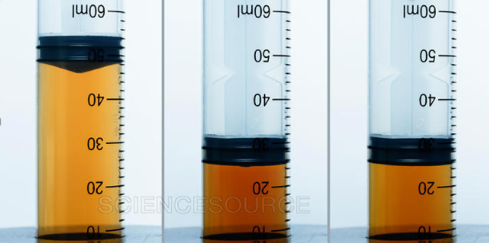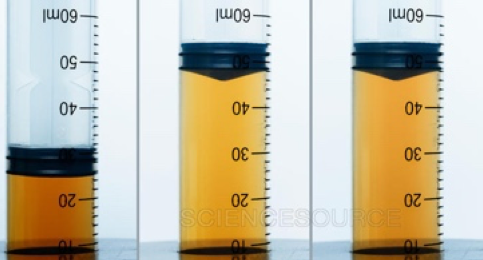# Nitrogen Dioxide & Dinitrogen Tetroxide Equilibrium

This is part of the HSC Chemistry course under the topic Factors that Affect Equilibrium

### HSC Chemistry Syllabus

• Conduct practical investigations to analyse the reversibility of chemical reactions

• Investigate the effects of temperature, concentration, volume and/or pressure on a system at equilibrium and explain how Le Chatelier’s principle can be used to predict such effects, for example:

– Interaction between nitrogen dioxide and dinitrogen tetroxide

### How does the nitrogen dioxide and dinitrogen tetroxide equilibrium work?

In this video, we will be exploring how nitrogen dioxide and dinitrogen tetroxide are able to be in equilibrium.

### Nitrogen Dioxide & Dinitrogen Tetroxide Equilibrium

• The equilibrium mixture of NO2 and N2O4 is investigated using a gas syringe (closed system).

$$2NO_{2(g)} \rightleftharpoons N_2O_{4(g)} \;\;\; \Delta H=-58 \; kJ mol^{-1}$$

• Nitrogen dioxide (NO2) is brown in colour while dinitrogen tetroxide (N2O4) is colourless

### Pressure & Volume• The effect of pressure and volume can be tested by pushing or pulling the plunger of the syringe to increase and decrease pressure respectively. Increasing the pressure directly increases the collision rate between gas molecules. This in turn increases the rate of reaction.Figure: volume of syringe decreases by half which in turn doubles concentration. Solution slowly becomes less brown as the chemical equilibrium shifts to the right (Le Chatelier’s principle).

• Pushing the plunger increases pressure and decreases volume. This increases the concentration of both gases. Solution initially turns brown quickly. However, since there are more moles of gases on the reactant side (NO2), the forward reaction rate is increased more than the reverse as a result of this change. By Le Chatelier’s principle, some of NO2 is consumed to form N2O4. This is represented by the gradual decrease in the solution’s brown appearance.

Increasing the pressure on the system favours the reaction that would produce less moles of gases. Chemical equilibrium shifts to the side with less moles of gases (N2O4) and solution turns less brown.Figure: volume of syringe doubles which in turn halves concentration. Solution slowly becomes browner as the chemical equilibrium shifts to the left (Le Chatelier’s principle).

• Pulling the plunger decreases pressure and increases volume. This decreases the concentration of both gases. Solution initially turns less brown quickly. The forward reaction rate is decreased more than the reverse as a result of this change. By Le Chatelier’s principle, some of N2O4 is consumed to form NO2. This is represented by the gradual increase in the solution’s brown appearance.

Reducing the pressure on the system favours the reaction that would produce more moles of gases. Chemical equilibrium shifts to the side with more moles of gases (NO2) and solution turns browner.

### TemperatureHeating the solution in warm water turns it brown as the formation of NO2 is endothermic.

• Heating the solution increases rate of reverse reaction (endothermic). This increases [NO2] and turns the solution browner.Icing the solution turns it pale as the formation of N2O4 is exothermic.

• Cooling the solution in ice reduces the rate of the reverse reaction (endothermic) more than the forward reaction. This means it favours the forward reaction (exothermic). This increases [N2O4] and causes the solution to turn pale.

Previous section: Practical Investigations of Le Chatelier's Principle (Iron (III) thiocyanate Equilibrium)

Next section: Practical Investigations of Le Chatelier's Principle (Combustion of magnesium and burning of steel wool